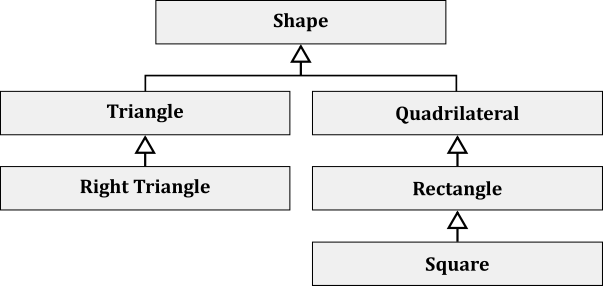# Quiz 8

## Wednesday

With a piece of paper, write down your name and answers to the following questions.

1. When a concrete class implements an interface, it must ________.

1. overload all of the methods listed in the interface
2. override all of the methods listed in the interface
3. not have any constructors
4. not have any fields
2. Abstract classes can ________.

1. be used as superclasses
2. be used as subclasses
3. have instance fields
4. All of the above
3. With the following inheritance hierarchy ...... and the following variable declarations ...

```Shape     shape     = new Shape();
Triangle  triangle  = new Triangle();
Rectangle rectangle = new Rectangle();
Square    square    = new Square();```

... which one of the following will cause a compiler error?

1. `shape = triangle`
2. `shape = square`
3. `square = null`
4. `square = rectangle`
4. What is an abstract class?

1. An abstract class is one without any derived classes.
2. An abstract class is any superclass with more than one subclass.
3. An abstract class is a class that contains abstract methods.
4. An abstract class is another name for “base class.”
5. What must be true if a derived class of an abstract superclass does not override all of the superclass’s abstract methods?

1. This is always an error on the subclass.
2. This is always an error on the superclass.
3. The derived class itself must be declared abstract.
4. The derived class is automatically non-abstract, and this is OK.

### Student Performance and Statistics

A histogram of student performance on percentage grades for Quiz 8 on Wednesday.

A table showing the average performance for each question in Quiz 8 on Wednesday.

## Friday

With a piece of paper, write down your name and answers to the following questions.

1. All classes directly or indirectly inherit from one class. What is this class’s name?

1. Super
2. Object
3. Root
4. Java
2. Fields in an interface are ________.

1. `final`
2. `static`
3. both `final` and `static`
4. not allowed
3. With the following inheritance hierarchy ...... which of the following array declarations is correct for an array that is expected to hold up to 10 objects of types `Shape`, `Rectangle`, and `Square`?

1. `Rectangle[] array = new Rectangle`
2. `Shape[] array = new Rectangle`
3. `Shape[] array = new Shape`
4. `Rectangle[] array = new Shape`
4. Here is an abstract method defined in a superclass:

`public abstract int sum(int[] arrary);`

Which of the following is required in a concrete subclass?

1. `public abstract int sum(int[] array) {...}`
2. `public int sum(int[] array) {...}`
3. `public double int sum(int[] array) {...}`
4. `public int sum(long[] array) {...}`
5. What is an abstract method?

1. An abstract method is any method in an abstract class.
2. An abstract method is a method that cannot be inherited.
3. An abstract method is declared with the reserved word `abstract`, and it does not have a method body.
4. An abstract method is a method in the derived class that overrids a superclass method.

### Student Performance and Statistics

A histogram of student performance on percentage grades for Quiz 8 on Friday.

A table showing the average performance for each question in Quiz 8 on Friday.